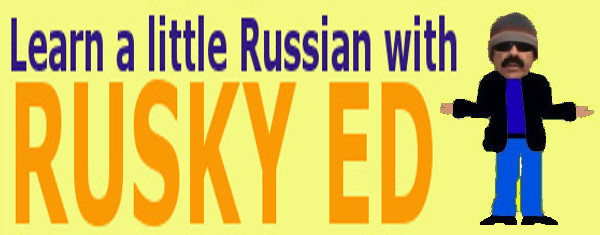Arithmetic - Miscellaneous
[ Number Sense ]    [ Addition and Subtraction ]    [ Multiplication and Division ]    [ Factors and Multiples ]    [ Decimal Operations ]    [ Fraction Operations ]    [ Miscellaneous ]    [ Home ]
 FEATURED VIDEOS:
```
```

## Arithmetic - Miscellaneous - Area of Rectangles

Area is the amount of space taken up by a shape. To find the area of a rectangle you multiply the width by the height. Consider this rectangle:This rectangle has a height of 13 units and a width of 14 units.

```
AREA = 13 x 14 = 182 square units

```
Remember units can be inches, feet, yards, meters, or any other unit of length. We use the term units when the actual unit is left unspecified.

To help you to visualize this a little better consider this example:As you can see this square contains 64 little squares. Each of these squares is a square unit. Area is measured in square units. This square has an area of 64 square units.

Here's another rectangle for you to take a look at.As you can see it has a height of 5 units and a length of 24 units.

```
AREA = 5 x 24 = 120 square units

```
Finding the area of a square is the same as for a rectangle.```
7 x 7 = 49 square units

```
You can find the area of other kinds of shapes, but finding the area of other shapes can be kind of tricky.
Custom Search
[ Number Sense ]    [ Addition and Subtraction ]    [ Multiplication and Division ]    [ Factors and Multiples ]    [ Decimal Operations ]    [ Fraction Operations ]    [ Miscellaneous ]    [ Home ]